Test: Discrete-Time Systems

# Test: Discrete-Time Systems - Electrical Engineering (EE)

Test Description

## 15 Questions MCQ Test GATE Electrical Engineering (EE) 2023 Mock Test Series - Test: Discrete-Time Systems

Test: Discrete-Time Systems for Electrical Engineering (EE) 2023 is part of GATE Electrical Engineering (EE) 2023 Mock Test Series preparation. The Test: Discrete-Time Systems questions and answers have been prepared according to the Electrical Engineering (EE) exam syllabus.The Test: Discrete-Time Systems MCQs are made for Electrical Engineering (EE) 2023 Exam. Find important definitions, questions, notes, meanings, examples, exercises, MCQs and online tests for Test: Discrete-Time Systems below.
Solutions of Test: Discrete-Time Systems questions in English are available as part of our GATE Electrical Engineering (EE) 2023 Mock Test Series for Electrical Engineering (EE) & Test: Discrete-Time Systems solutions in Hindi for GATE Electrical Engineering (EE) 2023 Mock Test Series course. Download more important topics, notes, lectures and mock test series for Electrical Engineering (EE) Exam by signing up for free. Attempt Test: Discrete-Time Systems | 15 questions in 45 minutes | Mock test for Electrical Engineering (EE) preparation | Free important questions MCQ to study GATE Electrical Engineering (EE) 2023 Mock Test Series for Electrical Engineering (EE) Exam | Download free PDF with solutions
 1 Crore+ students have signed up on EduRev. Have you?
Test: Discrete-Time Systems - Question 1

### x[n] and h[n] are given in the question. Compute the convolution y[n] = x[n] * h[n] and choose correct option. Que: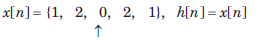Detailed Solution for Test: Discrete-Time Systems - Question 1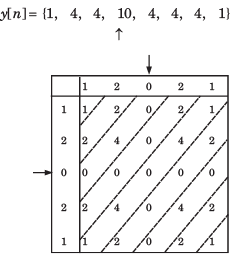Test: Discrete-Time Systems - Question 2

### x[n] and h[n] are given in the question. Compute the convolution y[n] = x[n] * h[n] and choose correct option. Que: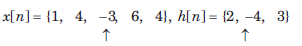Detailed Solution for Test: Discrete-Time Systems - Question 2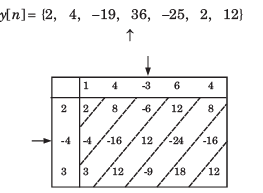Test: Discrete-Time Systems - Question 3

### x[n] and h[n] are given in the question. Compute the convolution y[n] = x[n] * h[n] and choose correct option.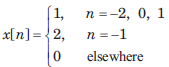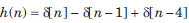Detailed Solution for Test: Discrete-Time Systems - Question 3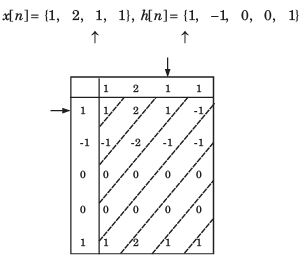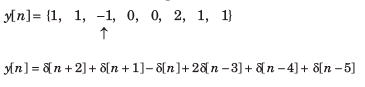Test: Discrete-Time Systems - Question 4

In question y[n] is the convolution of two signal. Choose correct option for y[n].Detailed Solution for Test: Discrete-Time Systems - Question 4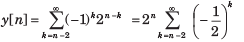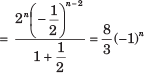Test: Discrete-Time Systems - Question 5

In question y[n] is the convolution of two signal. Choose correct option for y[n].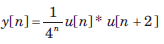Detailed Solution for Test: Discrete-Time Systems - Question 5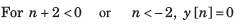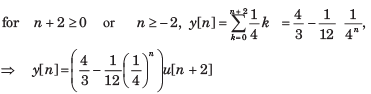Test: Discrete-Time Systems - Question 6

In question y[n] is the convolution of two signal. Choose correct option for y[n].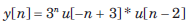Detailed Solution for Test: Discrete-Time Systems - Question 6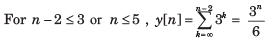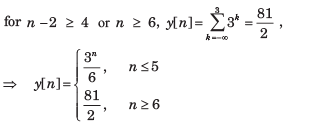Test: Discrete-Time Systems - Question 7

In question y[n] is the convolution of two signal. Choose correct option for y[n].

y[n] = u[n + 3] * u[n - 3]

Detailed Solution for Test: Discrete-Time Systems - Question 7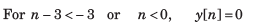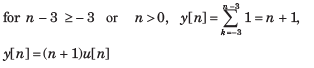Test: Discrete-Time Systems - Question 8

The convolution of x[n] = cos ( π/2 n) u[n] and h[n] = u[n - 1] is f [n]u[n - 1]. The function f [n] is

Detailed Solution for Test: Discrete-Time Systems - Question 8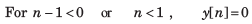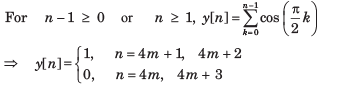Test: Discrete-Time Systems - Question 9

Let P be linearity, Q be time invariance, R be causality and S be stability. In question discrete time input x[n] and output y[n] relationship has been given. In the option properties of system has been given. Choose the option which match the properties for system.

Que: y[n] = rect (x[n])

Detailed Solution for Test: Discrete-Time Systems - Question 9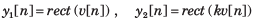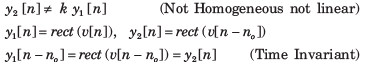At any discrete time n = no , the response depends only on the excitation at that discrete time. (Causal)

No matter what values the excitation may have the response can only have the values zero or one.

Test: Discrete-Time Systems - Question 10

Let P be linearity, Q be time invariance, R be causality and S be stability. In question discrete time input x[n] and output y[n] relationship has been given. In the option properties of system has been given. Choose the option which match the properties for system.

y[n] = nx[n]

Detailed Solution for Test: Discrete-Time Systems - Question 10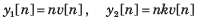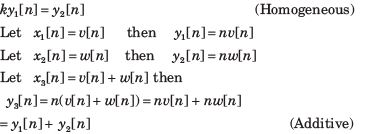Since the system is homogeneous and additive, it is also linear.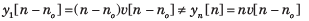At any discrete time, n = no the response depends only on the excitation at that same time. (Causal)

If the excitation is a constant, the response is unbounded as n approaches infinity. (Unstable)

Test: Discrete-Time Systems - Question 11

Let P be linearity, Q be time invariance, R be causality and S be stability. In question discrete time input x[n] and output y[n] relationship has been given. In the option properties of system has been given. Choose the option which match the properties for system.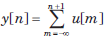Detailed Solution for Test: Discrete-Time Systems - Question 11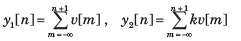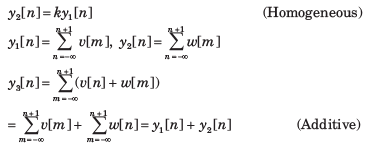Since the system is homogeneous and additive it is also linear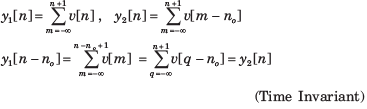At any discrete time, n =no , the response depends on the excitation at the next discrete time in future. (Anti causal)

If the excitation is a constant, the response increases without bound (Unstable)

Test: Discrete-Time Systems - Question 12

Let P be linearity, Q be time invariance, R be causality and S be stability. In question discrete time input x[n] and output y[n] relationship has been given. In the option properties of system has been given. Choose the option which match the properties for system.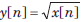Detailed Solution for Test: Discrete-Time Systems - Question 12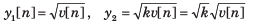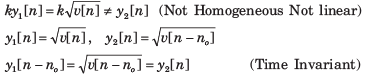At any discrete time n = no , the response depends only on the excitation at that time (Causal)

If the excitation is bounded, the response is bounded. (Stable).

Test: Discrete-Time Systems - Question 13

The output signal when a signal x(n)=(0,1,2,3) is processed through an ‘Delay’ system is:

Detailed Solution for Test: Discrete-Time Systems - Question 13

An delay system is a system whose output is same as the input, but after a delay.

Test: Discrete-Time Systems - Question 14

x[n] as shown in fig.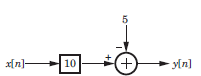Detailed Solution for Test: Discrete-Time Systems - Question 14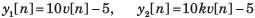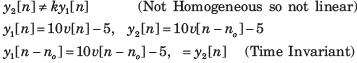At any discrete time, n = n0 the response depends only on the excitation at that discrete time and not on any future excitation. (Causal)

If the excitation is bounded, the response is bounded. (Stable).

Test: Discrete-Time Systems - Question 15

x[n] as shown in fig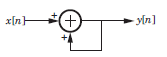Detailed Solution for Test: Discrete-Time Systems - Question 15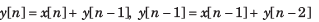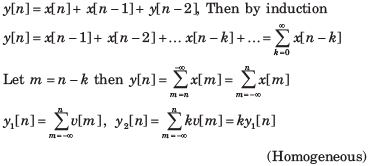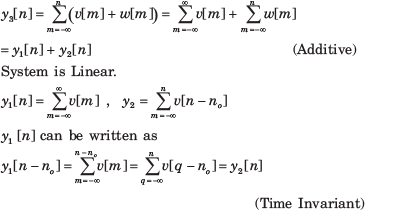At any discrete time n no the response depends only on the excitation at that discrete time and previous discrete time. (Causal)

If the excitation is constant, the response increase without bound. (Unstable)

## GATE Electrical Engineering (EE) 2023 Mock Test Series

22 docs|284 tests
Information about Test: Discrete-Time Systems Page
In this test you can find the Exam questions for Test: Discrete-Time Systems solved & explained in the simplest way possible. Besides giving Questions and answers for Test: Discrete-Time Systems, EduRev gives you an ample number of Online tests for practice

## GATE Electrical Engineering (EE) 2023 Mock Test Series

22 docs|284 tests(Scan QR code)